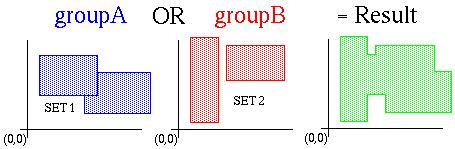Introduction Boolean algorithm
The input to the boolean operation routines consist of two sets of polygons. One set is the first operand of a boolean operation the other the second operand. What we get is the result of the boolean operation.

#The basic idea is to split the whole 2D plane in sections, called enclosed areas. The polygons in both sets divide the 2D plane in areas that are either of group A or group B or of both groups at the same time. A closed area is an area that can be the described by a simple polygon (not selfintersecting, not selfoverlapping). For the above example the enclosed areas would be like this

#The boolean result can be extracted, if we can tell for each enclosed area if it belongs to groupA, groupB are both groups. To be able to generate the enclosed area polygons we need to calculate the intersection of all polygon segments. So there are three major steps:
• calculate intersections for all polygons
• determine for each enclosed arae wether it belongs to groupA, groupB or both.
• extract the enclosed areas for a given boolean operation.